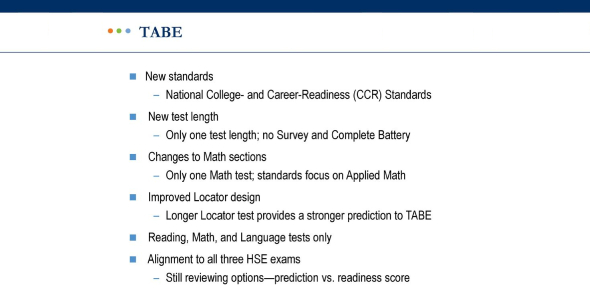# Quiz: TABE Computation Level M Trivia Test!

40 Questions | Attempts: 766
ShareSettings.

• 1.
297 + 333 =
• A.

620

• B.

621

• C.

603

• D.

602

• E.

None of These

• 2.
6,213 + 1,756 =
• A.

7,868

• B.

7,969

• C.

7,696

• D.

7,879

• E.

None of These

• 3.
596 + 7 =
• A.

604

• B.

603

• C.

593

• D.

693

• E.

None of These

• 4.
654 + 43 =
• A.

1,074

• B.

697

• C.

224

• D.

1,084

• E.

None of These

• 5.
8 +  6,584 =
• A.

6,682

• B.

6,592

• C.

6,584

• D.

6,594

• E.

None of These

• 6.
451 + 34 =
• A.

781

• B.

475

• C.

485

• D.

791

• E.

None of These

• 7.
79 - 25 =
• A.

45

• B.

64

• C.

55

• D.

53

• E.

None of These

• 8.
288 - 91 =
• A.

297

• B.

197

• C.

379

• D.

97

• E.

None of These

• 9.
853 - 150 =
• A.

703

• B.

903

• C.

953

• D.

753

• E.

None of These

• 10.
357 - 29 =
• A.

338

• B.

332

• C.

386

• D.

327

• E.

None of These

• 11.
4,356 - 187 =
• A.

4,128

• B.

4,178

• C.

4,187

• D.

4,278

• E.

None of These

• 12.
627 - 56 =
• A.

683

• B.

671

• C.

581

• D.

631

• E.

None of These

• 13.
7,137 × 4 =
• A.

28,448

• B.

29,548

• C.

28,528

• D.

28,428

• E.

None of These

• 14.
91 × 3 =
• A.

237

• B.

183

• C.

273

• D.

284

• E.

None of These

• 15.
18 × 356 =
• A.

6,408

• B.

5,968

• C.

5,308

• D.

9,808

• E.

None of These

• 16.
32 × 4 =
• A.

126

• B.

36

• C.

76

• D.

158

• E.

None of These

• 17.
43 ×17 =
• A.

700

• B.

711

• C.

731

• D.

374

• E.

None of These

• 18.
574 × 36 =
• A.

20,764

• B.

22,664

• C.

22,764

• D.

20,664

• E.

None of These

• 19.
61 × 13 =
• A.

793

• B.

244

• C.

733

• D.

693

• E.

None of These

• 20.
8,610 ÷ 7 =
• A.

132

• B.

1,130

• C.

1,230

• D.

123

• E.

None of These

• 21.
7 ÷ 5 =
• A.

1

• B.

1 R2

• C.

2

• D.

2 R1

• E.

None of These

• 22.
297 ÷ 9 =
• A.

30

• B.

23

• C.

32

• D.

31

• E.

None of These

• 23.
7 ÷ 3 =
• A.

2 R2

• B.

3 R1

• C.

2 R1

• D.

2 R3

• E.

None of These

• 24.
752 ÷ 8 =
• A.

92

• B.

84

• C.

86

• D.

94

• E.

None of These

• 25.
5 ÷ 3
• A.

1 R1

• B.

2 R1

• C.

2

• D.

1

• E.

None of These

## Related Topics

×

Wait!
Here's an interesting quiz for you.